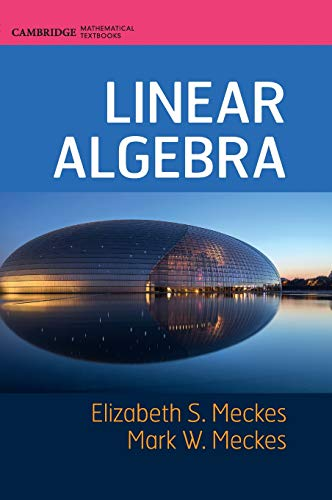# Linear Algebra (Cambridge Mathematical Textbooks)

4.5
Description

Linear Algebra offers a unified treatment of both matrix-oriented and theoretical approaches to the course, which will be useful for classes with a mix of mathematics, physics, engineering, and computer science students. Major topics include singular value decomposition, the spectral theorem, linear systems of equations, vector spaces, linear maps, matrices, eigenvalues and eigenvectors, linear independence, bases, coordinates, dimension, matrix factorizations, inner products, norms, and determinants.